Home    |    Teacher    |    Parents    |    Glossary    |    About UsEmail this page to a friendResources· Cool Tools · Formulas & Tables · References · Test Preparation · Study Tips · Wonders of MathSearchSpecial Functions (Math | Calculus | Integrals | Special Functions)Some of these functions I have seen defined under both intervals (0 to x) and (x to inf). In that case, both variant definitions are listed.
gamma = Euler's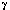constant = 0.5772156649...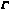(x) = Gamma(x) =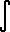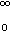t^(x-1) e^(-t)dt (Gamma function)
B(x,y) =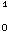t^(x-1) (1-t)^(y-1)DT
(Beta function)
Ei(x) =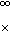e^(-t)/t DT (exponential integral) or it's variant, NONEQUIVALENT form:

Ei(x) =+ ln(x) +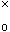(e^t - 1)/t DT = gamma + ln(x) +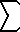(n=1..inf)x^n/(n*n!)
li(x) =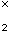1/ln(t) DT (logarithmic integral)
Si(x) =sin(t)/t DT (sine integral) or it's variant, NONEQUIVALENT form:
Si(x) =sin(t)/t DT = PI/2 -sin(t)/t DT

Ci(x) =cos(t)/t DT (cosine integral) or it's variant, NONEQUIVALENT form:
CI(x) = -COs(t)/t DT = gamma + ln(x) +(COs(t) - 1) / t DT (cosine integral)

Chi(x) = gamma + ln(x) +(cosh(t)-1)/t DT (hyperbolic cosine integral)
Shi(x) =sinh(t)/t DT (hyperbolic sine integral)
Erf(x) = 2/PI^(1/2)e^(-t^2) DT = 2/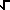PI(n=0..inf) (-1)^n x^(2n+1) / ( n! (2n+1) ) (error function)
FresnelC(x) =COs(PI/2 t^2) DT
FresnelS(x) =sin(PI/2 t^2) DT
dilog(x) =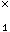ln(t)/(1-t) DT
Psi(x) =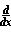ln(Gamma(x))
Psi(n,x) = nth derivative of Psi(x)
W(x) = inverse of x*e^x
L sub n (x) = (e^x/n!)( x^n e^(-x) ) (n) (laguerre polynomial degree n. (n) meaning nth derivative)
Zeta(s) =(n=1..inf) 1/n^s

Dirichlet's beta function B(x) =(n=0..inf) (-1)^n / (2n+1)^xTheorems with hyperlinks have proofs, related theorems, discussions, and/or other info.